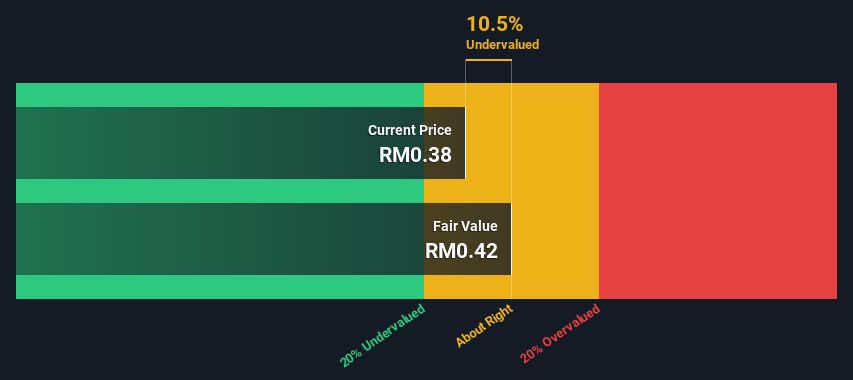Today we’ll do a simple run through of a valuation method used to estimate the attractiveness of South Malaysia Industries Berhad (KLSE:SMI) as an investment opportunity by taking the expected future cash flows and discounting them to their present value. The Discounted Cash Flow (DCF) model is the tool we will apply to do this. Don’t get put off by the jargon, the math behind it is actually quite straightforward.

Remember though, that there are many ways to estimate a company’s value, and a DCF is just one method. If you still have some burning questions about this type of valuation, take a look at the Simply Wall St analysis model.

See our latest analysis for South Malaysia Industries Berhad

### Step by step through the calculation

We use what is known as a 2-stage model, which simply means we have two different periods of growth rates for the company’s cash flows. Generally the first stage is higher growth, and the second stage is a lower growth phase. In the first stage we need to estimate the cash flows to the business over the next ten years. Seeing as no analyst estimates of free cash flow are available to us, we have extrapolate the previous free cash flow (FCF) from the company’s last reported value. We assume companies with shrinking free cash flow will slow their rate of shrinkage, and that companies with growing free cash flow will see their growth rate slow, over this period. We do this to reflect that growth tends to slow more in the early years than it does in later years.

A DCF is all about the idea that a dollar in the future is less valuable than a dollar today, so we discount the value of these future cash flows to their estimated value in today’s dollars:

#### 10-year free cash flow (FCF) estimate

 2022 2023 2024 2025 2026 2027 2028 2029 2030 2031 Levered FCF (MYR, Millions) RM5.41m RM5.70m RM5.98m RM6.25m RM6.51m RM6.77m RM7.04m RM7.30m RM7.57m RM7.85m Growth Rate Estimate Source Est @ 6.3% Est @ 5.48% Est @ 4.9% Est @ 4.49% Est @ 4.21% Est @ 4.01% Est @ 3.87% Est @ 3.78% Est @ 3.71% Est @ 3.66% Present Value (MYR, Millions) Discounted @ 10.0% RM4.9 RM4.7 RM4.5 RM4.3 RM4.0 RM3.8 RM3.6 RM3.4 RM3.2 RM3.0

(“Est” = FCF growth rate estimated by Simply Wall St)
Present Value of 10-year Cash Flow (PVCF) = RM39m

The second stage is also known as Terminal Value, this is the business’s cash flow after the first stage. For a number of reasons a very conservative growth rate is used that cannot exceed that of a country’s GDP growth. In this case we have used the 5-year average of the 10-year government bond yield (3.6%) to estimate future growth. In the same way as with the 10-year ‘growth’ period, we discount future cash flows to today’s value, using a cost of equity of 10.0%.

Terminal Value (TV)= FCF2031 × (1 + g) ÷ (r – g) = RM7.8m× (1 + 3.6%) ÷ (10.0%– 3.6%) = RM127m

Present Value of Terminal Value (PVTV)= TV / (1 + r)10= RM127m÷ ( 1 + 10.0%)10= RM49m

The total value, or equity value, is then the sum of the present value of the future cash flows, which in this case is RM88m. In the final step we divide the equity value by the number of shares outstanding. Compared to the current share price of RM0.4, the company appears about fair value at a 11% discount to where the stock price trades currently. The assumptions in any calculation have a big impact on the valuation, so it is better to view this as a rough estimate, not precise down to the last cent.KLSE:SMI Discounted Cash Flow May 16th 2022

### Important assumptions

We would point out that the most important inputs to a discounted cash flow are the discount rate and of course the actual cash flows. If you don’t agree with these result, have a go at the calculation yourself and play with the assumptions. The DCF also does not consider the possible cyclicality of an industry, or a company’s future capital requirements, so it does not give a full picture of a company’s potential performance. Given that we are looking at South Malaysia Industries Berhad as potential shareholders, the cost of equity is used as the discount rate, rather than the cost of capital (or weighted average cost of capital, WACC) which accounts for debt. In this calculation we’ve used 10.0%, which is based on a levered beta of 1.182. Beta is a measure of a stock’s volatility, compared to the market as a whole. We get our beta from the industry average beta of globally comparable companies, with an imposed limit between 0.8 and 2.0, which is a reasonable range for a stable business.